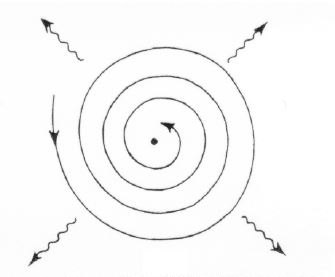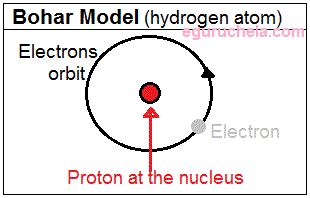Bohr Model of the Hydrogen Atom

In 1913 Niels Bohr introduced the atomic Hydrogen model which states that electrons orbit the nucleus at set distances. When an electron changes orbits, it does so in a sudden quantum leap. The energy difference between the initial and final orbit is emitted by the atom in bundles of electromagnetic radiation called photons. The Rutherford model had several flaws that the Bohr model overcame.

The Bohr model was based on his observations of the atomic emissions spectrum of the hydrogen atom. When white light is diffracted with a prism, all the colors of the visible spectrum can be seen. Each color corresponds to a specific amount of energy; however when the light given off by the hydrogen atom was passed through a prism, only certain colors of light could be seen. This led Bohr to theorize that electrons only have certain energies in an atom and they had to be in energy levels. Bohr found the energy of the colors of light that the hydrogen atom released. He used these energies to find the energies that the single electron in the hydrogen atom could have. Bohr said that the electron had to release energy to change its energy so the differences between the energies of light seen in the atomic spectrum should correspond to the differences in energies of the energy levels.

For better understanding of atomic, he bocombined classical theory with the early quantum concepts and gave his theory in three postulates:

1. Postulate I

In a radical departure from the established principles of classical mechanics and electromagnetism, Bohr stated that in an atom, the electron can move around in stable orbits without emitting radiant energy. Furthermore, he stated that each atom can exist in certain stable states.

2. Postulate II

Bohr defined these stable orbits in his second postulate as follows:

a. An electron revolves around the nucleus in orbits.

b. The angular momentum of revolution is an integral multiple of h/2p

where h = [6.6 x 10-34 J-s].

c. The angular momentum (L) of the orbiting electron is: L = nh/2p

3. Postulate III

In third postulate, Bohr incorporated early quantum concepts into the atomic theory. An electron can transition from a non-radiating orbit to another of a lower energy level. In doing so, a photon is emitted whose energy is equal to the energy difference between the two states. Therefore the frequency of the emitted photon is:

hv = Ei – Ef

where

Ei = energy of the initial state,

Ef = energy of the final state and Also Ei > Ef).

$$r_n = n^2 (\frac{h^2ε_0}{\pi m Ze^2})$$

where,

rn = radius of the nth orbit

n = integer

h = Planck’s constant

ε0 = Electric constant

m = Mass of the electron

Z = the Atomic number of the atom

e = Elementary charge

Since ε0, h, m, e, and p are constants and for a hydrogen atom Z = 1, rn α n2

The velocity of electron in Bohr’s stationary orbits

$$V_n = (\frac{Ze^2}{2hε_0})(\frac{1}{n})$$

Since ε0, h, & e are constants and for a hydrogen atom Z = 1, rn α n(1/n)

Total energy of electron in Bohr’s stationary orbits

$$E_n = -\frac{me^4}{8{ε_0}^2h^2} (\frac{Z^2}{n^2})$$ $$or E_n = -13.6 (\frac{Z^2}{n^2})cV$$

Negative sign (-) means that the electron is bound to the nucleus.Limitations of the Bohr Model of the Hydrogen Atom

Bohr’s model has problem with complex atoms and not able to explain following:

Why some spectral lines are more intense than others.

Why some spectral lines split into multiple lines in the presence of a magnetic field.

The Heisenberg’s uncertainty principle contradicts with Bohr’s idea of electrons existing in specific orbits with a known radius and velocity.

Importance of Bohr Model

It is important because it was the first model to postulate the quantization of electron orbits in atoms and it represents an early quantum theory that gave a start to developing modern quantum theory. It introduced the concept of a quantum number to describe atomic states.